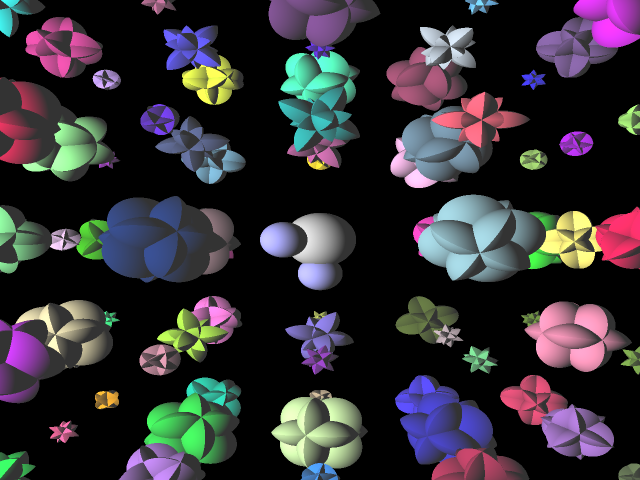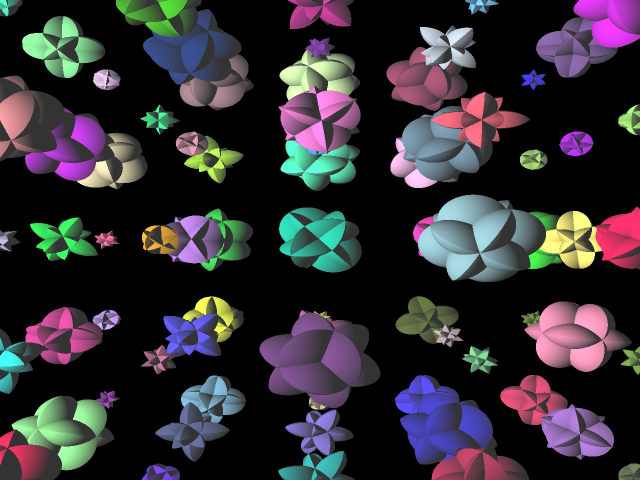# Raytracing 3D Distance Functions

#### Evan Ovadia, 570

In this project, I raytraced 3D distance functions. A 3D distance function can be thought of as a 3-dimensional array of floats. If the value in a cell is 0, that cell is considered "part of the surface". If the value in a cell is negative, that cell is considered inside the surface. If the value is positive, it's considered outside of the surface.

What makes this a 3D distance function instead of just a 3D function is that if a cell is positive, the value inside the cell is proportional to the distance to the surface. So for example, if the value in a cell is 3, that could mean that the nearest part of the surface is 3 cells away.

To raytrace this, we cast a ray, and if it hits the function's bounding box, then we start evaluating the 3D distance function. We sample at the edge of the box, where the ray hit. If the value is 9 there, for example, then we move the "current position" forward by 9 (note, it's not "towards the surface by 9", it's just forward by 9). Then, we sample again. Let's say it's 2 now, we now go forward by 2. We continue this process until we either hit the surface, or leave the bounding box.Usually, to visualize a 3D function like this, we would have to use marching cubes, and then smoothe the results, but this approach is much simpler and gives much more accurate results.Of course, this method is quite expensive, but not prohibitively so. Depending on how thin you consider the surface, there can be many iterations per ray. The best case is when the closest part of the surface is straight ahead, and the worst case is when the closest part of the surface is parallel to the ray.To raytrace the above 1280x960 image, it took 37 seconds.

The method is as flexible as any other method; one can add anti-aliasing and bloom to it easily.To raytrace the above 1280x960 image with 101x101 bloom and 4x4 anti-aliasing, it took 14 minutes, 33 seconds.

There are no regular numbers to compare it to though, because regular raytracers simply can't handle the same kind of input (3D distance functions).